# RRB clerk Prelims Quantitative Aptitude Quiz – 4

## RRB clerk Prelims Quantitative Aptitude Quiz

Quantitative aptitude measures a candidate’s numerical proficiency and problem-solving abilities. It is the most important section of almost all competitive exams. Candidates are often stymied by the complexity of Quantitative Aptitude Questions but if they practice more and more questions it will become quite easy. So, here we are providing you with the RRB Clerk Quantitative Aptitude Quiz to enhance your preparation for your upcoming examination. Questions given in this RRB Clerk Quantitative Aptitude Quiz are based on the most recent and the latest exam pattern. A detailed explanation for each question will be given in this RRB Clerk Quantitative Aptitude Quiz. This RRB Clerk Quantitative Aptitude Quiz is entirely free of charge. This RRB Clerk Quantitative Aptitude Quiz will assist aspirants in achieving a good score in their upcoming examinations.

Directions (1-5): Given below are the number of students (in hundreds) in two schools A and B, in different years. Answer the following questions based on given DI.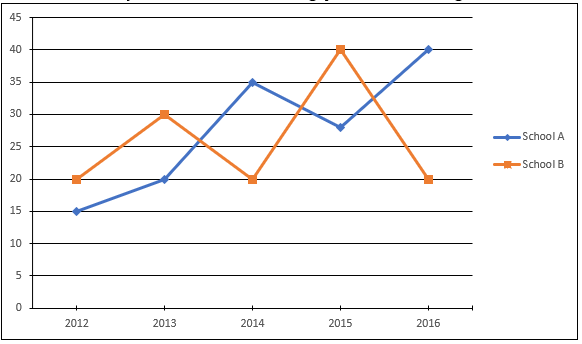1. What is the average number of students of school A in the year 2013, 2014 and 2016 and number of students of school B in the year 2012 and 2015 together?
(a) 3300
(b) 2500
(c) 3100
(d) 1550
(e) 22502. If in year 2017, number of students of school A decreases by 25% and that of school B increases by 10% as compared to year 2016. Find total number of students of school A and B together in 2017.
(a) 4500
(b) 5200
(c) 6500
(d) 4800
(e) 5000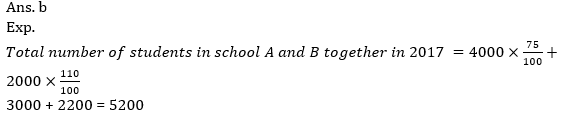3. Total number of students of school B in year 2015 is approximately what percent more than that of total number of students of school A in year 2012.
(a) 164%
(b) 162%
(c) 170%
(d) 172%
(e) 167%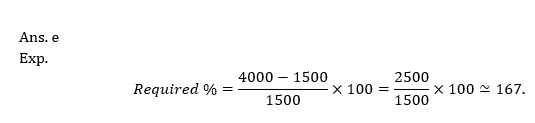4. If school A students in year 2012 decreases by 20% as compared to 2011. What was the total number of students in school A in 2011?
(a) 1875
(b) 1650
(c) 1700
(d) 1775
(e) 1675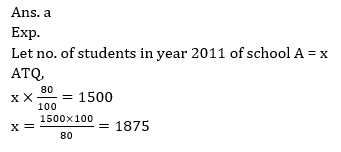5. Find the ratio of students in school A in the year 2013 and 2014 to the students in school B in the year 2015 and 2016 together?
(a)12 : 11
(b) 8 : 7
(c) 1 : 6
(d) 3 : 2
(e) 11 : 12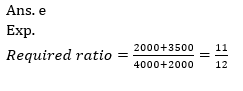6. How many 6 letter words can be formed using the word “MATTER” in which no T’s comes together?
(a) 600
(b) 300
(c) 340
(d) 260
(e) 240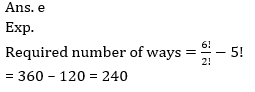7. Find the ratio of volume of cylinder ‘A’ to volume of cylinder ‘B’, if ratio of radius of cylinders ‘A’ to cylinder ‘B’ is 1 : 2 while the ratio of height of cylinder ‘A’ to cylinder ‘B’ is 2 : 1.
(a) 1 : 1
(b) 1 : 2
(c) 2 : 1
(d) 1 : 4
(e) 1 : 8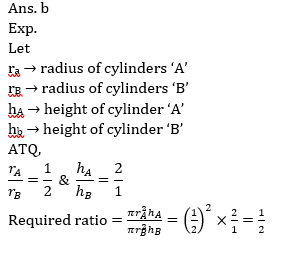8. A train moving at a speed of 70 m/s crosses a man moving at a speed of 5 m/s in opposite direction of train in 2 sec. Find the time taken by the train to cross another train of length 120 m travelling at speed of 55m/s in same direction.
(a) 15 sec.
(b) 10 sec.
(c) 12 sec.
(d) 18 sec.
(e) 20 sec.9. If 21 is added in a number, the result becomes 350/3% of itself. Find the new number?
(a) 126
(b) 147
(c) 130
(d) 136
(e)125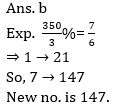10. Find the probability of choosing a team of 11 players from 8 batsmen and 8 bowler if it is given that number of batsman should always be greater the number of bowlers.
(a) 1/2
(b) 4/9
(c) 2/3
(d) 11/16
(e) 1/3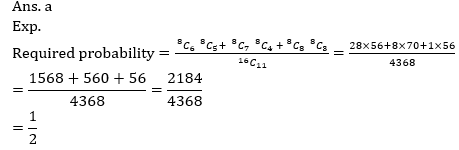###Click to Buy Bank MahaCombo PackageRecommended PDF’s for:

#### Most important PDF’s for Bank, SSC, Railway and Other Government Exam : Download PDF Now

AATMA-NIRBHAR Series- Static GK/Awareness Practice Ebook PDF Get PDF here
The Banking Awareness 500 MCQs E-book| Bilingual (Hindi + English) Get PDF here
AATMA-NIRBHAR Series- Banking Awareness Practice Ebook PDF Get PDF here
Computer Awareness Capsule 2.O Get PDF here
AATMA-NIRBHAR Series Quantitative Aptitude Topic-Wise PDF Get PDF here
AATMA-NIRBHAR Series Reasoning Topic-Wise PDF Get PDF Here
Memory Based Puzzle E-book | 2016-19 Exams Covered Get PDF here
Caselet Data Interpretation 200 Questions Get PDF here
Puzzle & Seating Arrangement E-Book for BANK PO MAINS (Vol-1) Get PDF here
ARITHMETIC DATA INTERPRETATION 2.O E-book Get PDF here

3